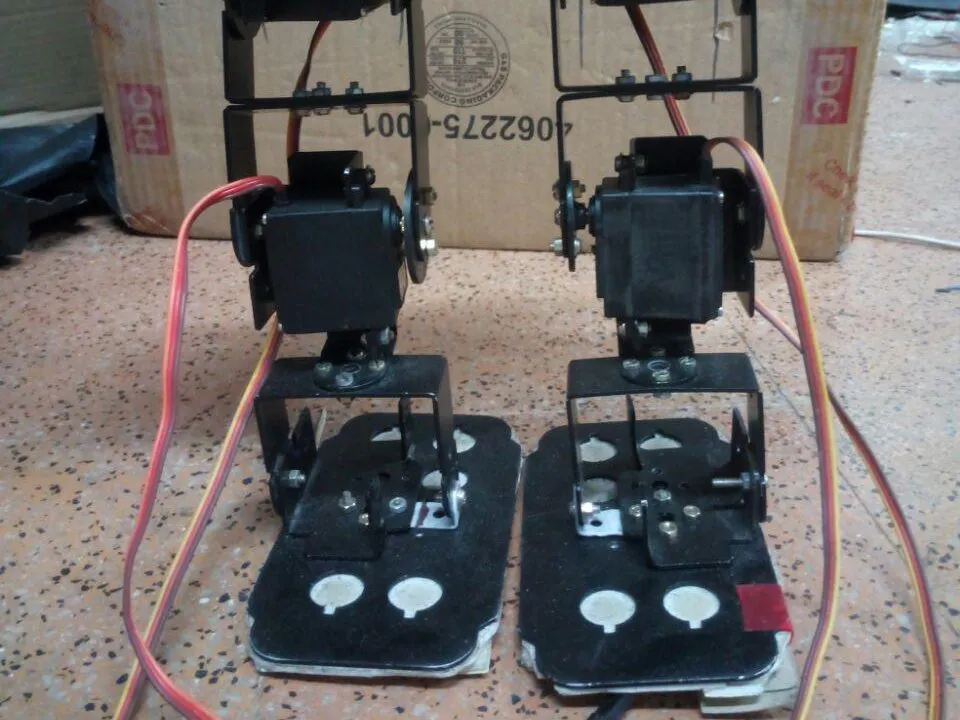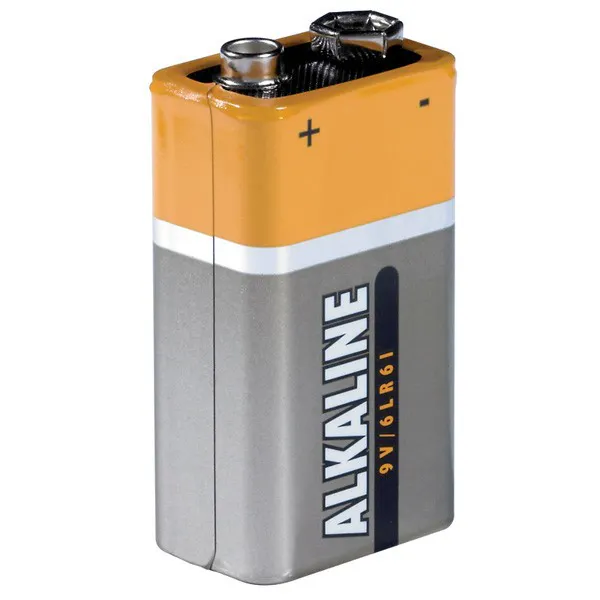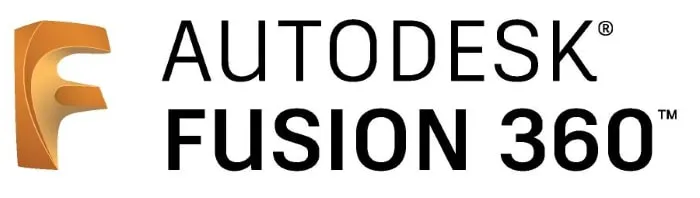Blynk Controlled BIPED

A biped controlled by using Blynk app.

AdvancedFull instructions providedOver 1 day1,285Things used in this project

Hardware components

 Servos (Tower Pro MG996R)
×6Jumper wires (generic)
×149V battery (generic)
×1
 Servo Shield
×1
 Double Side Tape
×1

Software apps and online servicesArduino IDEAutodesk Fusion 360

Hand tools and fabrication machines

 Driller

Custom parts and enclosures

The Design which we implemented.

Schematics

Servo Connection

Servo are Connected in this manner, based on which servo is assigned which pin on the shield. It is clearly mentioned on the program.

Code

Arduino Code

C/C++
This code is the final that gets uploaded to the arduino.
#include <Servo.h>
Servo a1,a2,b1,b2,c1;
int x;
String z;
char y;
int d=90;
int h=90;
int s=0,i=0,j=90,t=90;
int k=0,a=0,l=0,p=0,f=1,q=0,o=0;
long duration, distance;

void setup()
{
Serial.begin(9600);
a1.attach(11);
a2.attach(10);
b1.attach(9);
b2.attach(5);
c1.attach(3);
a1.write(90);
a2.write(90);
b1.write(90);
b2.write(90);
c1.write(95);
pinMode(12, OUTPUT);
pinMode(8, INPUT);
pinMode(2,OUTPUT);
digitalWrite(2,HIGH);
}

void loop()
{
if(Serial.available()>0)
{
y= z[z.length()-1];
}
digitalWrite(12, LOW);
delayMicroseconds(2);

digitalWrite(12, HIGH);
delayMicroseconds(10);

digitalWrite(12, LOW);
duration = pulseIn(8, HIGH);
distance = duration/58.2;
if(distance>=25||distance==0)
{
y=y;
}
else
{
y='s';
}
if(y=='f')
{
if(s==0)
{
// delay(5000);
c1.write(105);
delay(600);
while(k==0)
{
c1.write(105);
if(a==0)
{
k=1;
}
}
c1.write(95);
delay(100);
k=0;
}
for(i=90;i<=105;i+=1)
{
a1.write(i);
delay(30);
a2.write(j);
j=j-1;
delay(30);
}
delay(100);
for(i=90;i<=105;i+=1)
{
b1.write(i);
delay(30);
b2.write(t);
t=t-1;
delay(30);
}
delay(100);
c1.write(83);
delay(800);
while(k==0)
{
c1.write(83);
if(a==0)
{
k=1;
}
}
c1.write(95);
delay(200);
k=0;

c1.write(83);
delay(800);
while(k==0)
{
c1.write(83);
if(a==0)
{
k=1;
}
}
c1.write(95);
delay(100);
k=0;
for(i=x;i>=90;i-=1)
{
a1.write(i);
delay(30);
a2.write(x);
x=x+1;
delay(30);
}
delay(100);
for(i=x;i>=90;i-=1)
{
b1.write(i);
delay(30);
b2.write(x);
x=x+1;
delay(30);

}
delay(100);
for(i=90;i>=75;i-=1)
{
b1.write(i);
delay(30);
b2.write(d);
d=d+1;
delay(30);
}
delay(100);
for(i=90;i>=75;i-=1)
{
a1.write(i);
delay(30);
a2.write(h);
h=h+1;
delay(30);
}
c1.write(105);
delay(400);
while(k==0)
{
c1.write(105);
if(a==0)
{
k=1;
}
}
k=0;
c1.write(95);
delay(200);
c1.write(105);
delay(400);
while(k==0)
{
c1.write(105);
if(a==0)
{
k=1;
}
}
k=0;
c1.write(95);
delay(100);
for(i=x;i<=90;i++)
{
b1.write(i);
delay(30);
b2.write(x);
x=x-1;
delay(30);
}
delay(100);
for(i=x;i<=90;i++)
{
a1.write(i);
delay(30);
a2.write(x);
x=x-1;
delay(30);
}
delay(100);
/*c1.write(83);
delay(400);
while(k==0)
{
c1.write(83);
if(a==0)
{
k=1;
}
}
c1.write(95);
delay(100);*/
k=0;
d=90;
h=90;
t=90;
j=90;
s=1;
l=1;
f=0;
q=1;
}
//delay(50);
else if(y=='s')
{
if(l==1)
{
delay(100);
c1.write(83);
delay(800);
while(k==0)
{
c1.write(83);
if(a==0)
{
k=1;
}
}
c1.write(95);
delay(100);
k=0;
}
f=1;
p=0;
s=0;
l=0;
o=0;
q=0;
}
else if(y=='k')
{
if(f==1)
{
k=0;
c1.write(105);
delay(400);
while(k==0)
{
c1.write(105);
if(a==0)
{
k=1;
}
}
k=0;
c1.write(95);
delay(200);
}
f=0;

if(p==0)
{
/*c1.write(83);
delay(800);
while(k==0)
{
c1.write(83);
if(a==0)
{
k=1;
}
}
c1.write(95);
delay(100);
k=0;*/
for(i=x;i<=145;i++)
{
a1.write(i);
delay(30);
a2.write(x);
x=x-1;
delay(30);
}
delay(100);
//
for(i=x;i>=30;i--)
{
a2.write(i);
delay(30);
}

delay(100);

for(i=x;i>=100;i--)
{
a1.write(i);
delay(30);
}
delay(100);
for(i=x;i<=105;i++)
{
b1.write(i);
delay(60);
b2.write(x);
x=x-1;
delay(60);
}
delay(100);
for(i=x;i>=55;i--)
{
a1.write(i);
delay(30);
}
delay(100);
for(i=x;i<=100;i++)
{
a1.write(i);
delay(30);
}
delay(100);
for(i=x;i>=90;i--)
{
b1.write(i);
delay(60);
b2.write(x);
x=x+1;
delay(60);
}
delay(200);
for(i=x;i<=145;i++)
{
a1.write(i);
delay(30);
}

delay(100);
for(i=x;i<=35;i++)
{
a2.write(i);
delay(30);
}
delay(100);
for(i=x;i>=90;i-=1)
{
a1.write(i);
delay(30);
a2.write(x);
x=x+1;
delay(30);
}
delay(100);
//
c1.write(83);
delay(800);
while(k==0)
{
c1.write(83);
if(a==0)
{
k=1;
}
}
c1.write(95);
delay(100);
k=0;
p=1;
l=0;
delay(50);
}
}
else if(y=='l')
{
if(q==1)
{
c1.write(83);
delay(800);
while(k==0)
{
c1.write(83);
if(a==0)
{
k=1;
}
}
c1.write(95);
delay(100);
k=0;
}
q=0;
delay(100);
if(o==0)
{
for(i=x;i<=105;i+=1)
{
b1.write(i);
delay(30);
b2.write(x);
x=x-1;
delay(30);
}
delay(3000);
for(i=x;i>=90;i--)
{
b1.write(i);
delay(30);
b2.write(x);
delay(30);
x=x+1;
}
delay(100);
}
o=1;
}
}

Credits

Akash Ravichandran

13 projects • 75 followers
Developer and a Learner